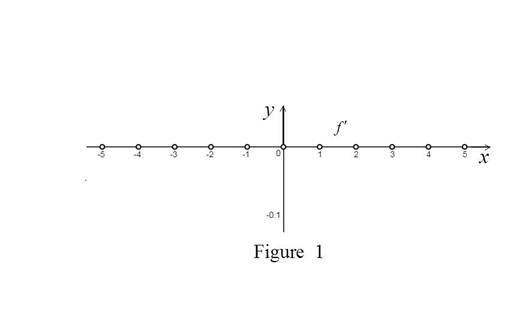# The points where the greatest integer function f ( x ) = 〚 x 〛 is not differentiable and then to find the formula for f ′ and sketch the graph.### Single Variable Calculus: Concepts...

4th Edition
James Stewart
Publisher: Cengage Learning
ISBN: 9781337687805### Single Variable Calculus: Concepts...

4th Edition
James Stewart
Publisher: Cengage Learning
ISBN: 9781337687805

#### Solutions

Chapter 2.7, Problem 52E
To determine

## To find: The points where the greatest integer function f(x)=〚x〛 is notdifferentiable and then to find the formula for f′ and sketch the graph.

Expert Solution

The greatest integer function is not differentiable at x

The formula for the derivative function is f(x)={0if xundefinedif x.

### Explanation of Solution

Result Used:

The greatest integer function is defined as follows,

f(x)={y   if x:y is nearest interger less than xx   if x

Calculation:

Obtain the points where the greatest integer function f(x)=x is not differentiable.

The derivative of the greatest integer function is calculated as follows,

Case 1: x is not an integer.

f(x)=limh0f(x+h)f(x)h=limh0x+hxh

Since x is not an integer, x+h=x.

f(x)=limh0xxh=limh00h=0

Thus, f(x)=0

Case 2: x is an integer.

The left hand derivative of the function is computed as follows,

f(x)=limh0f(x+h)f(x)h=limh0x+hxh

Since h approaches 0 from left, x+h<x.

This implies that, x+h=x1.

limh0x+hxh=limh0x1xh=1limh0h=10

Thus, the left hand derivative of the function does not exist. This follows that, f(x) does not exist.

Therefore, the greatest integer function is not differentiable at the integer points.

By case (1) and (2), it is defined that the formula for the derivative is f(x)={0if xundefinedif x.

Graph:

Use the above information and trace the graph of f as shown below in Figure 1,From the Figure 1, it is clear that the greatest integer function is discontinuous at integer points.

### Have a homework question?

Subscribe to bartleby learn! Ask subject matter experts 30 homework questions each month. Plus, you’ll have access to millions of step-by-step textbook answers!